Courses

# Fracture Concepts Civil Engineering (CE) Notes | EduRev

## Civil Engineering (CE) : Fracture Concepts Civil Engineering (CE) Notes | EduRev

The document Fracture Concepts Civil Engineering (CE) Notes | EduRev is a part of Civil Engineering (CE) category.
All you need of Civil Engineering (CE) at this link: Civil Engineering (CE)

Fracture Concepts
→ Fracture mechanics:
o Failure of concrete structures typically involves crack propagation and growth of large cracking zones before the maximum load is reached. Fracture mechanics, for design of concrete structures, has been introduced for a realistic prediction of crack stability.
o Some reasons for introducing fracture mechanics into the design of concrete structures:
1. Energy required for crack formation,
2. The need to achieve objectivity of finite element solutions,
3. Lack of yield plateau,
4. The need to rationally predict ductility and energy absorption capability, and
5. The effect of structure size on the nominal strength, ductility, and energy absorption capability.

o Fracture problem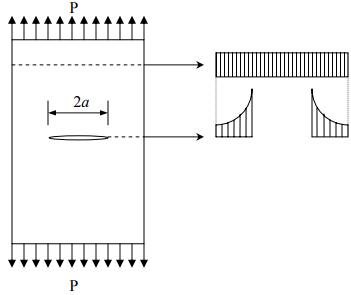→ The focus of the problems in fracture mechanics is to study the stress distribution around the crack and the propagation of the crack.
→ Cracks are prescribed geometrically in the material.
→ Energy-based crack propagation (failure) criterion is usually applied in the approach.
→The method allows investigation of the following phenomena during the propagation of cracks:
1. Strain-softening due to distributed cracking,
2. Localization of cracking into large fractures prior to failure, and
3. Bridging stresses at the fracture front.
→ Traditionally, the size effect has been explained by statistical theories, in terms of the randomness of strength distribution.

→ Weibull’s statistical theory (Weibull’s weakest link statistics)
o Weibull-type theory of failure
The probability of failure of a structure under load P and the mean normal stress at failure are: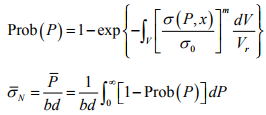where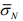= mean normal stress,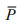= mean load,
V = volume of structure,
Vr = representative volume of material,
m = Weibull modulus of the material,
σ0 = scaling parameter, and
σ (P x, ) = a function representing the stress caused by load P at point x.

→ The Weibull-type statistical explanations of the size effect cannot be applied to concrete structures because:
1. It ignores the size effect caused by the redistribution of stress prior to failure, and
2. It ignores the consequent energy release from the structure.

o Assumptions of the brittle failure of concrete structures
1. The propagation of a fracture or crack band requires an approximately constant energy supply per unit area of fracture plane.
2. The potential energy released by the structure due to the propagation of the fracture or crack band is a function of both the fracture length and the size of the fracture process zone at the fracture front.
3. The failure modes of geometrically similar structures of different sizes are also geometrically similar.
4. The structure does not fail at crack initiation. o Maximum stress while the crack is propagating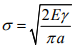where E = Young’s modulus, 2a = characteristic length of the crack, γ = surface energy. r is the function of material property and crack geometry.

o Cracking modes: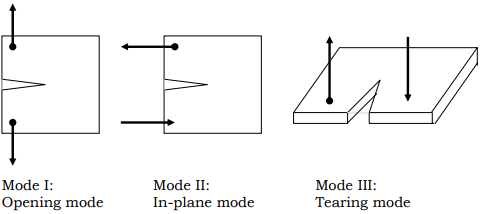Linear elastic fracture mechanics (LEFM)
o Assumptions:
1. All of the fracture process happens at the crack tip, and
2. The entire volume of the body remains elastic.
→ Under these assumptions, crack propagation and structural failure can be investigated by methods of linear elasticity.

o Stress singularity
In a sufficiently close neighborhood of the sharp crack tip, the stress components are the same regardless of the shape of the body and the manner of loading.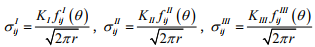where I, II, III refer to the elementary modes,
θ = the polar angle,
K, KII , KIII = stress intensity factors, and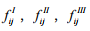functions are the same regardless of the body geometry and the manner of loading.

o Energy criterion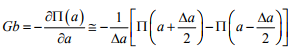where Π = U-W = potential energy of the structure,
U = strain energy of the structure as a function of the crack length a, and
W = work of loads.

o Critical energy release rate
Define G = energy release rate and Gc = critical energy release rate,
→ the crack is stable if the calculated G < Gc,
→ the crack is propagating if the calculated G > Gc, and
→ the crack is in meta stable equilibrium if the calculated G = Gc.

Suppose a structure with an interior crack (existing crack) with thickness b, the energy released by crack growth is calculated as

Gb∆a = P∆x − ∆Ue
where = ∆Uchange in elastic energy due to crack growth . ∆a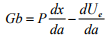Introduce the compliance function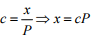The strain energy Ue is given by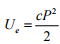thus,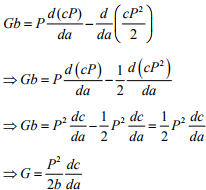→ Gccorresponds to that at fracture. The method is known as “critical energy release rate” method.
o Stress intensity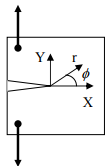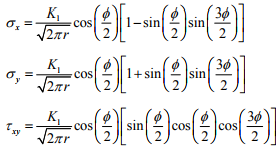where K1 is stress intensity factor for Mode I. Dimensional analysis shows that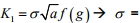applied stress, a = characteristic length f( g) = a function representing geometrical properties.

o Calculation of Gi
For Model I,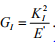For Model II,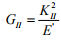For Model III,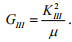where E' = E = Young’s modulus, and  � = elastic shear modulus. For the case of plane strain,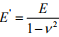where ν = Poisson’s ratio.

→ For general loading, the total energy release rate is G=GI + GII + GIII

o Calculation of KI
The stress intensity factor Kcan be calculated from σij (or τij ) expressions or from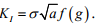Also,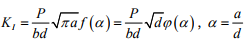where α = the relative crack length,
d = characteristic structure dimension, and
ϕ (a ) = f(a )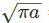= a nondimensional function.

→ For geometrically similar structures of different sizes, the stress intensity factor is proportional to the square root of the size, and the energy release rate is proportional to the size of the structure.
→ The condition of mode I crack propagation is as follow:
K= KK
where K= GFE' critical value of ' KI = fracture toughness

→ The nominal stress at failure is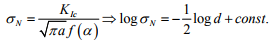→ The size effect plot according to linear elastic fracture mechanics is an inclined straight line of slope of -1/2.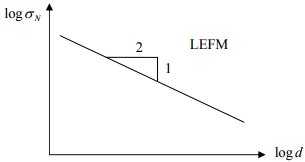o Fracture process zone
→ In concrete, microcracks develop ahead of crack tip creating a “fracture process zone”. Characteristics of this zone is of fundamental importance in the development of nonlinear fracture mechanics of concrete. Experimental methods are continuously developed.
→ In the HSC the tensile strength can be 2-5 times greater than NSC. However, the increase in fracture energy or elastic modulus is not much. Consequently, HSC may be brittle.

o Applicability of LEFM:
→ In fracture mechanics, the fracture process zone must be having some finite size. The size is characterized by material properties, such as the maximum aggregate size .d→ The length and effective width of the fracture process zone of concrete in three-point bend specimens are roughly 12da and 3da , respectively.
→ LEFM is applicable when the length of the fracture process zone is much smaller than the cross section dimension of the structure, which is not satisfied for most of the concrete structures.
→ Brittleness → Brittleness is the function of fracture energy.
→ Nonhomogeneity of cracked concrete

→ Fictitious crack model
o Developed by Hillerborg to capture the complex nature of concrete in tension.
o Up to the peak, the change in length due to crack propagation is assumed to be a linear function of the strain. ∆L = Lε
After peak a localized fracture develops. There is a softening behavior inside the fracture zone. This is called strain localization. However, in the region near crack tip (localization of deformation), this assumption is not valid. The modified form for ∆L is thus:
∆L = Lε + w
where w = the term representing the influence of localization in fracture zone.
o Therefore, two relationships are needed to characterize the mechanical behavior:
1. σ −ε relationship for region outside the fracture zone.
2. σ −w relationship for the fracture zone.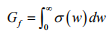This is determined experimentally using notched specimens.

Offer running on EduRev: Apply code STAYHOME200 to get INR 200 off on our premium plan EduRev Infinity!

,

,

,

,

,

,

,

,

,

,

,

,

,

,

,

,

,

,

,

,

,

;